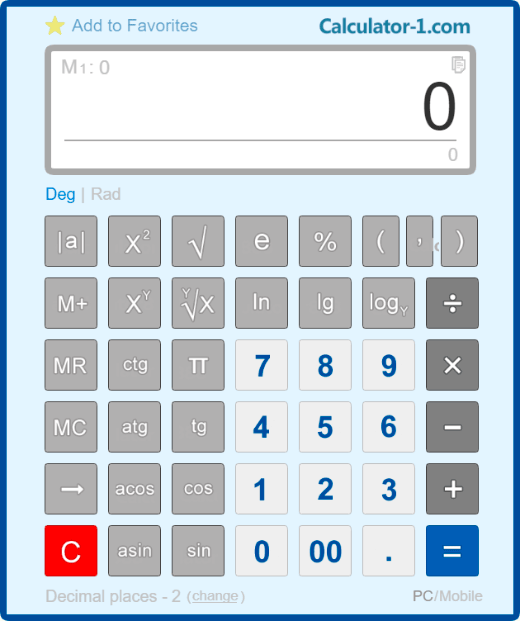# Scientific CalculatorA scientific calculator supports calculations of trigonometric functions, such as sine, cosine, tangent, cotangent, etc. Using this calculator, you can find the logarithm of a number, raise to a power, find a root square and, moreover, a nth root.

A scientific calculator is easy to use online-there is no need to download or install it on your PC or laptop.

For any calculation, you can use either mouse or numpad.

Moreover, you can customize the settings of the calculator and save them.

Below you can find examples and information on how to use a scientific calculator.

## How to work with a scientific calculator

### Calculator keys to perform trigonometric functions

[ sin ] - means sine of an angle;
[ cos ] - means cosine of an angle;
[ tg ] - means tangent of an angle;
[ ctg ] - means cotangent of an angle;
[ π ] - is a mathematical constant, the ratio of a circle’s circumference to its diameter;
[ e ] - is a mathematical constant, Euler’s number;
[ log ] - is the common logarithm;
[ ln ] - is the natural logarithm (to the base e);
[ logY ] - is the logarithm of X to the base Y;
[ √ ] - calculates the square root;
[ X2 ] - raises X to the power of 2;
[ XY ] - raises X to the power of Y.

### Functions of the standard buttons

[ 0 ], [ 1 ], [ 2 ], ... [ 9 ] - are standard numeric keys;
[ 00 ] - is the key for entering 2 zeros;
[ → ] - deletes the last character entered on the display;
[ +/- ] - changes the mathematical sign of the displayed number to the opposite one;
[ + ] - addition, [ - ] - subtraction, [ х ] - multiplication, [ ÷ ] - division;
[ % ] - calculates percentages;
[ M+ ] - saves the number to memory with the [ + ] sign;
[ M- ] - saves the number to memory with the [ - ] sign;
[ MR ] - displays the memory content;
[ MC ] - erases the memory content;
[ AC ] - reset the calculator and reset the memory;
[ C ] - resets the calculator without resetting the memory.

### Entering commands using a computer keyboard

The calculator supports input from any numeric keys of a computer keyboard: both the numeric keys on the top and the separate numeric keypad on the right.

You can use the [Enter] key to enter the equal character.
To delete the last character, press the [Backspace] (arrow) key.
To enter a plus sign, use the [ + ] key at the top or on the side numeric keypad.
To enter a minus sign, use the [ - ] key at the top or on the side numeric keypad.
Use the [ * ] key on the side numeric keypad to enter the multiplication sign.
Use the [ / ] key on the side numeric keypad to enter the division sign.
You can use the [Esc] and [Del] keys on top of the keyboard, or the [End] key on the side numeric keypad to reset the calculator.

### Examples of calculations performed using the online calculator

Tangent of a 45 degrees angle: 45 [ tg ]. The result is 1.
Sine of a 30 degrees angle: 30 [ sin ]. The result is 0.5.
The common logarithm of 100: 100 [ log ]. The result is 10.
The logarithm of 125 to the base 5: 125 [ logY ] 5. The result is 3.
Calculating the square root of 529: 529 [ √ ]. The result is equal to 23.
Raising 3 to the power of 4: 3 [ XY ] 4 [ = ]. The result is equal to 81.
Calculating a percentage of a number: 500 [ х ] 25 [ % ]. The result is equal to 125.
Calculating what percent is one number of another number: 25 [ ÷ ] 500 [ % ]. The result is equal to 5(%).
Adding a percentage to a number: 500 [ + ] 25 [ % ]. The result is equal to 625.
Subtracting a percentage from a number: 500 [ - ] 25 [ % ]. The result is equal to 375.

### History of calculators

The direct predecessor of a calculator is a mechanic and portable counting machine called arithmometer. It was primary used for multiplication and division. Though it is possible to use arithmometer for addition or subtraction, it is quite difficult to do. Based on its working principle, the predecessor of a calculator is a digital device, that is why the result of calculation is always one hundred percent correct.